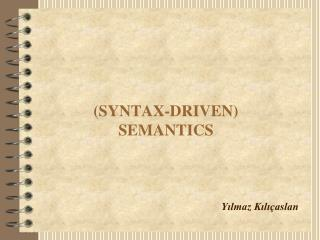# (SYNTAX-DRIVEN) SEMANTICS - PowerPoint PPT PresentationDownload Presentation(SYNTAX-DRIVEN) SEMANTICS

(SYNTAX-DRIVEN) SEMANTICSDownload Presentation## (SYNTAX-DRIVEN) SEMANTICS

- - - - - - - - - - - - - - - - - - - - - - - - - - - E N D - - - - - - - - - - - - - - - - - - - - - - - - - - -
##### Presentation Transcript

1. (SYNTAX-DRIVEN)SEMANTICS Yılmaz Kılıçaslan

2. Outline • Semiotic Triangle • Syntax • Semantics • Pragmatics • (Syntax-driven) Semantics • Semantics via languages of logic • Model-based semantics • Lambda-calculus • Computational Issues • Coding the semantic value of basic expressions • Handling the lambda operator • Implementing compositionality with lambda calculus

3. Semiotic Triangle • The three branches of semiotics: • syntactics(syntax)—how signs relate to other signs (example: how the word “dog” relates to the other words in the sentence “The dog ate my homework.”) • semantics—study of how signs relate to things (example: how the word “dog” relates to an actual dog) • pragmatics—actual use of codes in everyday life; effects of signs on human behavior and how people mold signs and meanings in their actual interaction (example: How would the sentence “The dog ate my homework” be used in everyday life? How would my teacher react to it?) Ogden and Richard’s Semiotic Triangle

4. A Formal Language for First-Order Logic SYNTAX: SEMANTICS: (Ignoring Quantification) MODEL: 0 1 1 0 0 1 0 1 Con   Quant   |  []M = M = <A, F> A = {a, b, c} Term  Name Term  Var F(j) = aF(v) = c F(k) = b F(m) =c 0 1 0 1 0 1 Name  j | k | v | m Var  x | y | z | x1 |... []M = Pred1 S Pred2  L For  Pred1(Term) For  Pred2(Term, Term) For  [For Con For] For   For For  Quant Var For [Pred1(α)]M = F(Pred1)(F(Term)) a b c 0 1 a b c F(S) = [Pred2(α,β)]M = [F(Pred2)(F(β))](F(α)) a b c a b c a b c [F1  F2]M= [[]M([F1]M)]([F2]M) 0 1 0 1 0 1 [ F]M= []M([F]M) F(L) = Example: [[L(j, k)]]M = []M([L(j, k)]]M) = []M([F(L)(F(k))](F(j))) = []M(0) = 1 = []M([F(L)(b)](a))

5. Natural Language Semantics in FOL Compositional Translation: Example:̏John loves Katy̋ S L(j,k) N VP xL(x,k) John j V N Katy k loves yxL(x,y)

6. A Fatal Problem with Semantics in FOL Referentially Opaque Constructions: i.e. constructions creating contexts whereLeibnitz’ Law fails. The result of substituting in any formula one name for another name denoting the same individual results in a formula that is true iff the original formula was true Example: (a)̏John believes that the Morning Star is the Morning Star.̋ (b)̏John believes that the Morning Star is Venus.̋ [m]M = [v]M = c ⇒ [B(j, m= m)]M = [B(j, m= v)]M But, (b) might be false while (a) is true. B(j, m= m) B(j, m= v)

7. Intensional Logic (IL) A Platonic View of Reality Plato’s Ideas Frege’s Senses Carnap’s Intensions Kripke’s Possible Worlds Montague’s Intensional Logic SYNTAX: SEMANTICS: MODEL: F(j) = a a . . . For  (Term = Term) . . . Expr  ^Expr . . . M = <A, W, F> A = {a,b,c,d} W = {w1,w2} ⇔ [α]M,w = [β]M,w [α = β]M,w = 1 F(m) = F(v) = w1 w2 c c w1 w2 [^α]M = w1 w2 c d Example: [^(m = m)]M = [^(m = v)]M = w1 ... wN w1 w2 w1 w2 1 1 1 0 [α]M,w1 [α]M,wN

8. Natural Language Semantics in IL Translations into IL: De Dicto Readings: (a)̏John believes that the Morning Star is the Morning Star.̋ (b)̏John believes that the Morning Star is Venus.̋ A De Re Reading: ̏John believes that the Morning Star is Venus.̋ B(j, ^(m = m)) B(j, ^(m = v)) [^(m = m)]M [^(m = v)]M As ≠ (b) can be false while (a) is true. x[B(j, ^(x = v))](m)

9. Problems with Possible Worlds Semantics • Logically Equivalent Sentences : • If two sentences and are logically equivalent, then it will always be a logically valid inference from B(α, ^) to B(α, ^), for any believer denoted by α. • However, a person might believe a sentence without believing its equivalent. Example: Necessarily True Sentences For every rational believer denoted by α, the following would hold: B(α, ^(m = m)) But, B(α, ^(ex = )) could still be false.

10. Conclusion Using formal languages of logic as a bridge, we can develop a truth-conditional, model-theoretic natural language semantics adhering to the principle of compositionality. However, this approach ultimately fails (e.g. in the case oflogically equivalent sentences in opaque contexts).